# Constructing Images of the Objects

 Page 11/14 Date 23.04.2018 Size 1.62 Mb. #45829

## Constructing Images of the Objects

If we have functions to clothe the framed vectors with images of the vertebrae, then we could re-create their image from the framed vectors that have just been computed. For instance, if the image were a ring, it might be written as follows.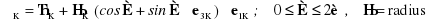This expression states to move to the center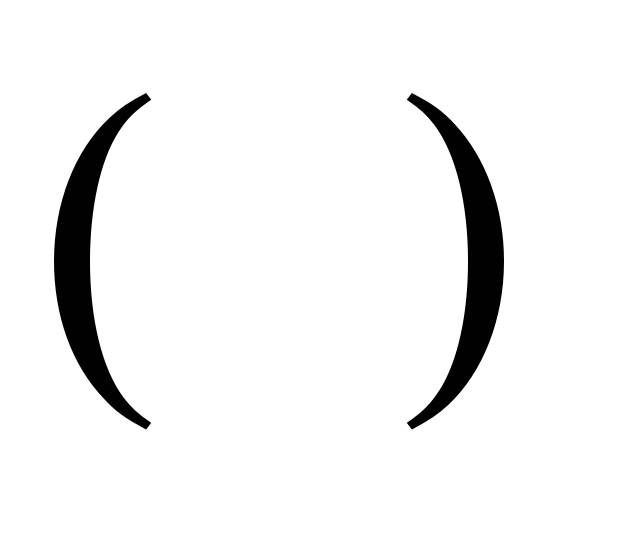of the K’th object and then rotate a vector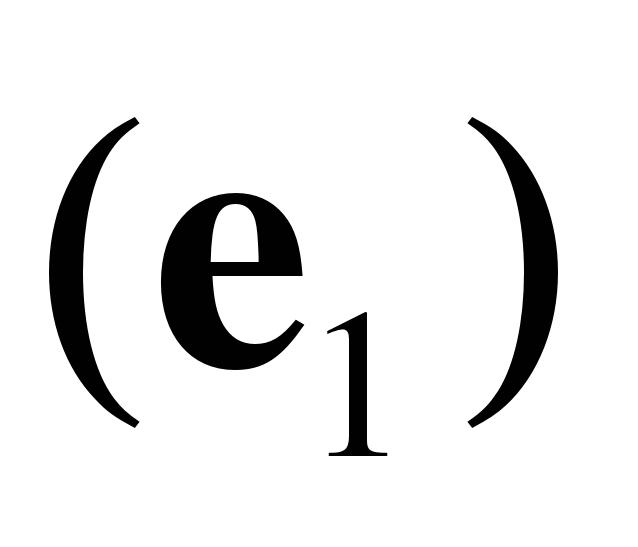of lengthabout that center in the plane perpendicular to the third element of the vertebra’s orientation frame of reference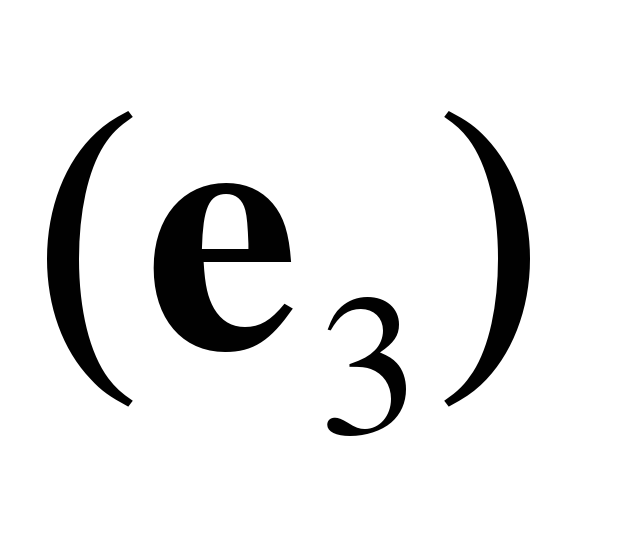through an angular excursion of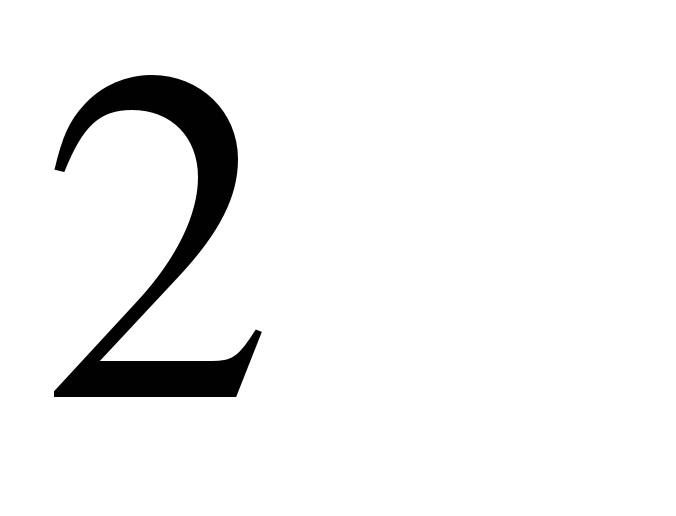radians. The rotating vector traces out a circle centered upon the center of the K’th object.

If we want it to be a torus, then we can draw a series of circles about the curve that has just been defined.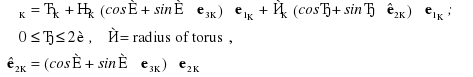This expression says that, for every element of the circle just described, construct the tangential to the circle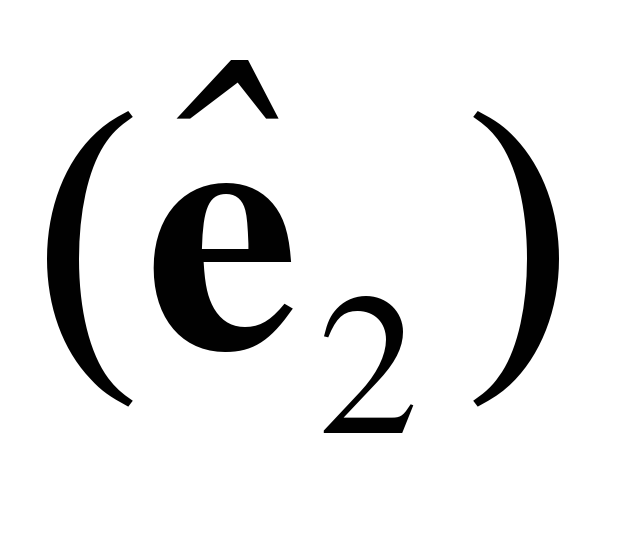and the radial vector, which is multiplied by the scalar, and then rotate the radial vector about the circle through an angular excursion ofradians.

It is also easy to plot the axes of rotation in their correct location. The location of the N’th axis of rotation for the K’th object is given by the following relationship.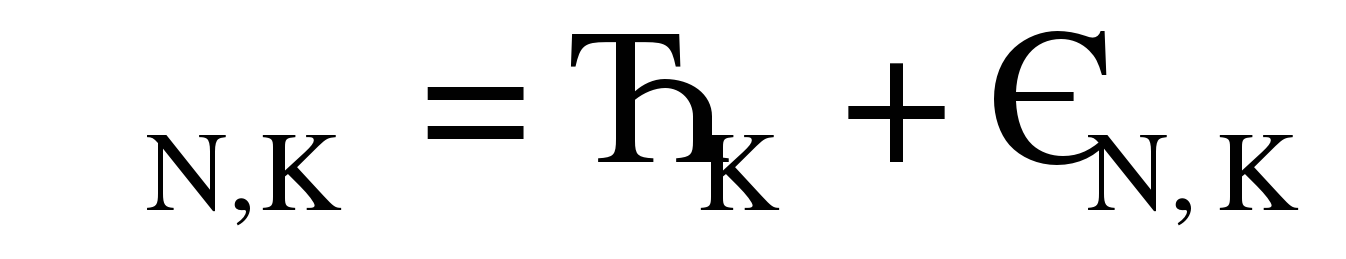The direction of the N’th axis of rotation is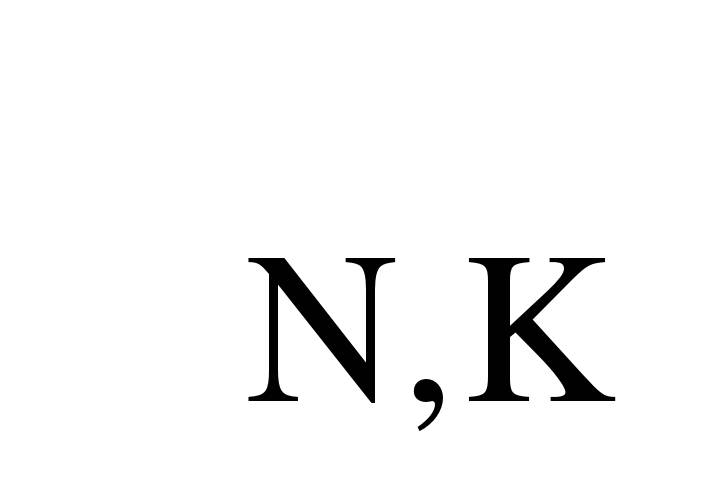.